# Optimization Algorithms in Deep Learning

In this blog we are going to study about various optimization algorithms . We would answer several questions like , what was the need to study several other optimization algorithms other than the algorithms we used to solve Machine Learning optimization problems ? Which are the important optimization algorithms used in Deep Learning ? We would come to know which algorithm to choose when ? And in the end we would learn how to implement our code in keras .

## Why we need advanced optimizers ?

We used batch gradient descent, batch Stochastic gradient descent and simple gradient descent algorithms to solve optimization problems . For a loss function L(w) we may have following possibilities:

The slope at maxima, minima and saddle point is 0. The loss function of Logistic regression, linear regression and SVM only contain a minima or a maxima but no saddle points because the function is convex . Saddle points are present in the loss function of Neural Networks because the function is complex . So we need some better optimization problem solving algos.

Using SGD if we get stuck with a local minima or a saddle point, we ll not get out of it because the gradient term becomes zero. So the w_new gets equal to the w_old. It all depends on how we initialize w. We ll get different minima for different initializations.

If we initialize hill descent in 3d we get contours. Basically we get a number for each contour. If the number increases from outer contour to inner contour we say it is a local maxima and if the number decreases from outer contour to inner contour we say it is a local minima.

## Normal SGD and its drawbacks

SGD stochastically approximates the gradient that would be generated using GD. Which is faster depends a lot on the size of the data. As using GD would require fewer iterations than SGD but each iteration in SGD is faster as we are only using a subset of points.

Noisy update is not because of the noisy data points. It is because we are not taking the gradient of each datapoint and then updating the weight. Instead we are taking the average of gradient over a batch size. That is why we are getting the noisy updates. Using SGD the gradient shoots itself in different directions. Its like playing ping pong where if you have only 2 sticks, your ball would take a long time to reach to the upper hole because you have very few points to control the trajectory of the ball. But if you have multiple sticks in multiple stages, you have more power to control the ball from different positions and your ball would not travel all around the table.

## Batch SGD with Momentum

In SGD we updated our weights using the gradient term of the current iteration, but when we use momentum we add the exponentially weighted gradient term of the previous iteration to the current gradient term to update the weights. In this way our algorithm learns from its previous iterations too.

This technique also helps in denoising. The new updates that are formed using the momentum are denoised . Essentially, when using momentum, we push a ball down a hill. The ball accumulates momentum as it rolls downhill, becoming faster and faster on the way (until it reaches its terminal velocity if there is air resistance, i.e. γ<1). The same thing happens to our parameter updates: The momentum term increases for dimensions whose gradients point in the same directions and reduces updates for dimensions whose gradients change directions. As a result, we gain faster convergence and reduced oscillation.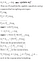0 < γ≤ 1 . So as the power of gamma increases the value decreases i.e. lesser weight is given to the momentum of previous iterations.

In this update we don’t update the weights (w_old) using momentum and gradient at w_old in the current iteration simultaneously, but we update the current weights(w_old) first using momentum to get an intermediate location(w’), now we update these current intermediate weights using the gradients calculated on these intermediate weights, to get the final updated weights (w_new).

However, a ball that rolls down a hill, blindly following the slope, is highly unsatisfactory. We’d like to have a smarter ball, a ball that has a notion of where it is going so that it knows to slow down before the hill slopes up again.

When we move towards a minimum w we might overstep from the actual minimum because of the exponentially weighted sum + gradient of the previous point.

1. SGD with Nesterov Accelerated Gradient : We have now crossed the minimum after moving in the direction of momentum; so by finding gradient at this point (slope is positive) so we need to move in opposite direction so faster convergence by finding gradient at momentum( we will reach to momentum earlier than SGD + momentum).
2. SGD with momentum: We will move more distance than the NAG , which will make oscillations , which causes convergence to be slow ( eventually we ll reach to the solution but might take more iterations).

In SGD and SGD + momentum we had a learning rate same for each weight. But some features in the dataset are sparse(BoW) and some are dense (w2v). When our data has sparse features, keeping or having a single learning rate for all of our weights is not good for our optimization. So in AdaGrad we are going to have an adaptive learning rate i.e. one weight for each parameter.

To do this AdaGrad algos dynamically incorporate knowledge of the geometry of the data observed in past iterations, it then applies that knowledge to set lower learning rates for more frequently occurring features and higher learning rates for relatively infrequent occurring features. As a result the infrequent features stand out, enabling the learner to identify rare, yet highly predictive features .

RMSProp = It is just Adadelta with default value of γ set to 0.95 . Else everything remains the same .This value is pre-defined in keras.

Adam is another algorithm which uses adaptive learning rates to update the weights. It is one of the most famous optimizers of 2018.

m_t and v_t are estimates of the first moment (the mean) and the second moment (the uncentered variance) of the gradients respectively, hence the name of the method. As m_t and v_t are initialized as vectors of 0’s, the authors of Adam observe that they are biased towards zero, especially during the initial time steps, and especially when the decay rates are small (i.e. β1 and β2 are close to 1).

Using eda on g_t has been discussed to be beneficial when we discussed SGD with momentum . So we can think of Adam as a culmination of the ideas where we are using eda of both g_t and squared g_t to speed up the convergence.

## Optimizers in keras

Its been enough of theory and maths , so now let’s jump to the code. We ll be dealing with MNIST dataset . You can download the dataset from here. MNIST dataset is a simple computer vision dataset . It consists of 28 X 28 pixel images of handwritten digits . The dataset has 784 dimensions. There are 10 class labels from 0 to 9.

Now we’ll define our layer architecture. We ll be using simple Deep MLP to build our architecture with 3 hidden layers and a softmax layer.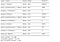This is the summary of our architecture which shows the account of parameters in our architecture.

First we ll be using SGD as our optimizer.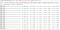Here we have chosen SGD optimizer to train our model and then we are fitting the model on train data and then testing it using test data. We have trained the model using 12 epochs . The time taken along with the loss and accuracy score is written along with each epoch in the above code snippet.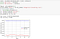Now we plot the epoch vs loss plot .

We observe that there is large difference between the validation loss and the train loss and 12 epochs are not enough to get the optimum result using SGD optimizer.

Now we ll be using RMSProp optimizer.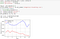RMSProp doesn’t make much difference even. It ll require more epochs to reach the minma.

Now we ll use Adam optimizer.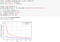So we can observe that when we are using Adam optimizer we are requiring lesser epochs to reach the minima and there is not much gap between the train and validation loss.

From above observations we can conclude that Adam optimizer is the best optimizer so far because it reaches its minima in very less number of epochs as compared to other optimizers . SGD is the slowest optimizer .

## Which optimizer to choose when ?

Choosing SGD , SGD + momentum and NAG as optimizers won’t help us in sparse data . And these optimizers take more number of epochs , thus more time to train , and if we have a large dataset we may have to wait for huge amount of time for just our models to train. SGD is a simple optimizer which works well for convex functions but doesn’t equally work well for the complex functions like in Deep NN , this is because in complex functions we may get stuck at saddle points and local minima and SGD is not capable of taking us out of that.

So choosing an adaptive learning rate method is better than choosing simple SGD or modified SGD. It helps us reach the minima in feawer epochs than simple SGD.